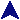Using Arguments ICode Examples```#
# Some fairly random examples of
# subroutines, using different features.
#

use strict;

#
# redund(n, str) returns the string str
# concatinated to itself n times.
# This uses a standard technique for
# functions of a fixed number of arguments.
# (The perl x operator will do this.)
#
sub redun
{
# This is like a parameter list.
my(\$rptct, \$str) = @_;

if(\$rptct < 1) { return ""; }

my(\$result) = "";
while(\$rptct--) {
\$result .= \$str;
}

return \$result;
}

#
# glueem(a, b, c, d, ...) takes any number
# of arguments and returns the concatination
# of them all.  This uses the array of arguments
# as an array to produce a variadic function.
#
sub glueem
{
my(\$result) = "";
my(\$arg);
foreach \$arg (@_) {
\$result = "\$result\$arg";
}

return \$result;
}

#
# readall(fn) attempts to read the named
# file and return its contents as a single
# string.  If it is successful, it returns
# the list (1, contents).  If not, it
# returns (0, errmsg).  Returning a list is really
# a way of having more than one return value.
#
{
my(\$fn) = @_;

open(IN, \$fn) or return (0, \$!);

my(\$line);
my(\$result) = "";
while(\$line = <IN>) {
\$result .= \$line;
}

return (1, \$result);
}

#
# rev(list) reverses the contents of a list
# and returns the new list.  There is a builtin
# function reverse which already does this.
#
sub rev
{
my(@arr) = @_;
my(\$low, \$hi) = (0, \$#_);

while(\$low < \$hi) {
my(\$temp) = \$arr[\$low];
\$arr[\$low] = \$arr[\$hi];
\$arr[\$hi] = \$temp;
++\$low;
--\$hi;
}

return @arr;
}

# Some repeated runduancy.
my \$argle = redun(5, "smith ");
print "\$argle\n";
\$argle = redun(2, "verily ");
print "\$argle\n\n";

# All to one.  (Parens are optional in calls.)
\$argle = glueem "Lets ", "see ", "if ", "this ", "works.";
print "\$argle\n";
\$argle = glueem ("Seems", " to.");
print "\$argle\n\n";

# Can you read me?
my \$fn = "f1.txt";
my (\$ok, \$text) = readall(\$fn);
if(\$ok) {
print "File \$fn is:\n\$text\n";
} else {
print "File \$fn open failed: \$text.\n";
}
\$fn = "bogus.txt";
(\$ok, \$text) = readall(\$fn);
if(\$ok) {
print "File \$fn is:\n\$text\n\n";
} else {
print "File \$fn open failed: \$text.\n";
}

# Suffering some reversals?
my @mike = ("How", "are", "you?");
my @sam = rev @mike;
print "@sam\n";
my \$bill = glueem rev("this?", "see ", "you ", "Do ");
print "\$bill\n";

# And, it makes a handy swap function, too.
\$argle = 10;
my \$bargle = 72;
(\$argle, \$bargle) = rev(\$argle, \$bargle);
print "\$argle \$bargle\n";
```